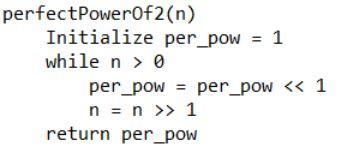# Smallest perfect power of 2 greater than n (without using arithmetic operators)

Given a non-negative integer n. The problem is to find the smallest perfect power of 2 which is greater than n without using the arithmetic operators.

Examples :

```Input : n = 10
Output : 16

Input : n = 128
Output : 256
```

## Recommended: Please try your approach on {IDE} first, before moving on to the solution.

Algorithm :## C++

 `// C++ implementation of smallest perfect power ` `// of 2 greater than n ` `#include ` ` `  `using` `namespace` `std; ` ` `  `// Function to find smallest perfect power ` `// of 2 greater than n ` `unsigned ``int` `perfectPowerOf2(unsigned ``int` `n) ` `{ ` `    ``// To store perfect power of 2 ` `    ``unsigned ``int` `per_pow = 1; ` ` `  `    ``while` `(n > 0)  ` `    ``{ ` `        ``// bitwise left shift by 1 ` `        ``per_pow = per_pow << 1; ` ` `  `        ``// bitwise right shift by 1 ` `        ``n = n >> 1; ` `    ``} ` ` `  `    ``// Required perfect power of 2 ` `    ``return` `per_pow; ` `} ` ` `  `// Driver program to test above ` `int` `main() ` `{ ` `    ``unsigned ``int` `n = 128; ` `    ``cout << ``"Perfect power of 2 greater than "` `        ``<< n << ``": "` `<< perfectPowerOf2(n); ` `    ``return` `0; ` `} `

## Java

 `// JAVA Code for Smallest perfect ` `// power of 2 greater than n ` `import` `java.util.*; ` ` `  `class` `GFG { ` `     `  `    ``// Function to find smallest perfect ` `    ``// power of 2 greater than n ` `    ``static` `int` `perfectPowerOf2( ``int` `n) ` `    ``{ ` `        ``// To store perfect power of 2 ` `         ``int` `per_pow = ``1``; ` `      `  `        ``while` `(n > ``0``)  ` `        ``{ ` `            ``// bitwise left shift by 1 ` `            ``per_pow = per_pow << ``1``; ` `             `  `            ``n = n >> ``1``; ` `        ``} ` `      `  `        ``// Required perfect power of 2 ` `        ``return` `per_pow; ` `    ``} ` `     `  `    ``// Driver program ` `    ``public` `static` `void` `main(String[] args)  ` `    ``{ ` `         ``int` `n = ``12``; ` `         ``System.out.println(``"Perfect power of 2 greater than "` `                            ``+ n + ``": "` `+ perfectPowerOf2(n)); ` `        ``} ` `    ``} ` `         `  `    ``//This code is contributed by Arnav Kr. Mandal. `

## Python3

 `# Python3 implementation of smallest  ` `# perfect power of 2 greater than n ` ` `  `# Function to find smallest perfect  ` `# power of 2 greater than n ` `def` `perfectPowerOf2( n ): ` `     `  `    ``# To store perfect power of 2 ` `    ``per_pow ``=` `1` `     `  `    ``while` `n > ``0``: ` `     `  `        ``# bitwise left shift by 1 ` `        ``per_pow ``=` `per_pow << ``1` `         `  `        ``# bitwise right shift by 1 ` `        ``n ``=` `n >> ``1` `         `  `    ``# Required perfect power of 2 ` `    ``return` `per_pow ` ` `  `# Driver program to test above ` `n ``=` `128` `print``(``"Perfect power of 2 greater than"``, ` `            ``n, ``":"``,perfectPowerOf2(n)) ` ` `  `# This code is contributed by "Sharad_Bhardwaj". `

## C#

 `// C# Code for Smallest perfect ` `// power of 2 greater than n ` `using` `System; ` ` `  `class` `GFG { ` ` `  `    ``// Function to find smallest perfect ` `    ``// power of 2 greater than n ` `    ``static` `int` `perfectPowerOf2(``int` `n) ` `    ``{ ` `        ``// To store perfect power of 2 ` `        ``int` `per_pow = 1; ` ` `  `        ``while` `(n > 0)  ` `        ``{ ` `            ``// bitwise left shift by 1 ` `            ``per_pow = per_pow << 1; ` ` `  `            ``n = n >> 1; ` `        ``} ` ` `  `        ``// Required perfect power of 2 ` `        ``return` `per_pow; ` `    ``} ` ` `  `    ``// Driver program ` `    ``public` `static` `void` `Main() ` `    ``{ ` `        ``int` `n = 128; ` `        ``Console.WriteLine(``"Perfect power of 2 greater than "` `+ ` `                           ``n + ``": "` `+ perfectPowerOf2(n)); ` `    ``} ` `} ` ` `  `// This code is contributed by Sam007 `

## PHP

 ` 0)  ` `    ``{ ` `        ``// bitwise left shift by 1 ` `        ``\$per_pow` `= ``\$per_pow` `<< 1; ` ` `  `        ``// bitwise right shift by 1 ` `        ``\$n` `= ``\$n` `>> 1; ` `    ``} ` ` `  `    ``// Required perfect power of 2 ` `    ``return` `\$per_pow``; ` `} ` ` `  `    ``// Driver code ` `    ``\$n` `= 128; ` `    ``echo` `"Perfect power of 2 greater than "``.  ` `          ``\$n` `. ``": "``.perfectPowerOf2(``\$n``); ` ` `  `// This code is contributed by mits  ` `?> `

Output:

```Perfect power of 2 greater than 128: 256
```

Time Complexity: O(num), where num is the number of bits in the binary representation of n.

Don’t stop now and take your learning to the next level. Learn all the important concepts of Data Structures and Algorithms with the help of the most trusted course: DSA Self Paced. Become industry ready at a student-friendly price.

My Personal Notes arrow_drop_upCheck out this Author's contributed articles.

If you like GeeksforGeeks and would like to contribute, you can also write an article using contribute.geeksforgeeks.org or mail your article to contribute@geeksforgeeks.org. See your article appearing on the GeeksforGeeks main page and help other Geeks.

Please Improve this article if you find anything incorrect by clicking on the "Improve Article" button below.

Improved By : Mithun Kumar

Article Tags :
Practice Tags :

Be the First to upvote.

Please write to us at contribute@geeksforgeeks.org to report any issue with the above content.